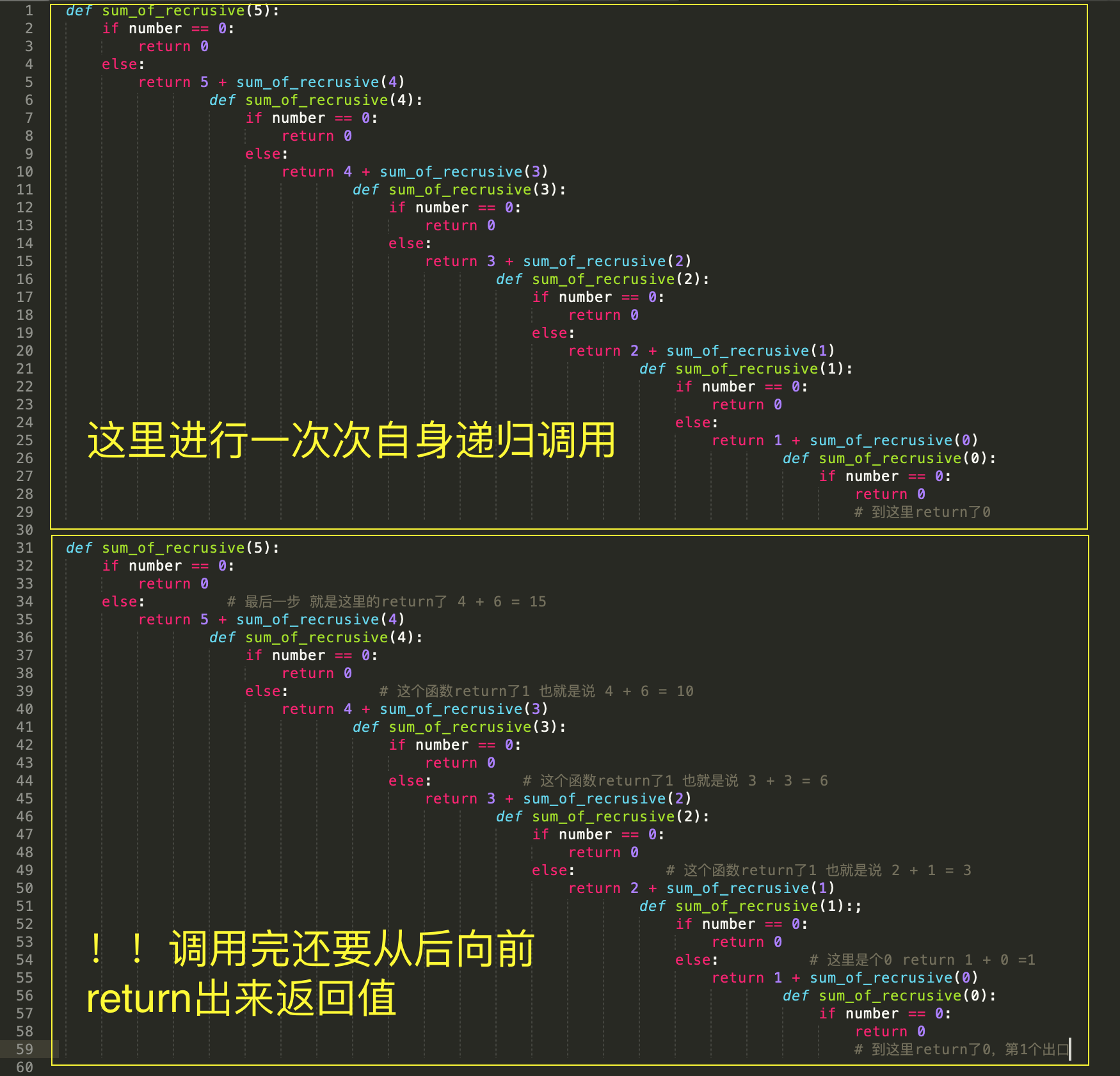## 1. 基本问题Q&A

### 1-2 迭代和递归有什么区别？

```  # 迭代实现
def iterative_of_factorial(n):
result = 1
for i in range(2,n+1):
result *= i
return result
print(iterative_of_factorial(5))

# 递归实现
def recursive_of_factorial(n):
if n == 1:
return 1
else:
return n * recursive_of_factorial(n-1)
print(recursive_of_factorial(5))

# 多看几遍可以看出来，递归最后return的是自身。而迭代通过的是不断重复运行下一个函数。
```

### 1-3 递归Code的时候要注意什么

a. 自己调用自己
b. 必须有结束条件作为递归出口，不然死循环了。

## 2. 实现一个简单递归并解析

```def sum_of_recrusive(number):
if number == 0:
return 0
else:
return number + sum_of_recrusive(number-1)

print(sum_of_recrusive(5))
# result
# 15
```## 3. 实现稍微复杂的递归

1. 找规律
2. 找条件
3. 查漏补缺(主要是考虑各种极值问题，比如0，1这种特殊情况)

### 3-1 斐波那契数列

```# 递归写法，给列表个数返回列表

def fibonacci_of_rec(number: int) -> list:
"""1.递归实现"""
if number == 1:
return 0
elif number == 2:
return 1
else:
return fibonacci_of_rec(number-1) + fibonacci_of_rec(number-2)

def print_fib(number: int) -> list:
"""2.打印"""
fib_list = []
for i in range(1,number+1):
fib_list.append(fibonacci_of_rec(i))
return fib_list

print(print_fib(10))
# resule
# [0, 1, 1, 2, 3, 5, 8, 13, 21, 34]

# 迭代写法，给数字范围返回列表

def fibonacci_of_iter(n: str) -> list:
fib_list = []
a, b = 0, 1
while a < n:
fib_list.append(a)
a, b = b, a + b
return fib_list

print(fibonacci_of_iter(1000))

# result
# [0, 1, 1, 2, 3, 5, 8, 13, 21, 34, 55, 89, 144, 233, 377, 610, 987]
```

### 3-2 汉诺塔

```def move(n: int, a: str, b, c):
if n == 1:
print('move', a, '-->', c)
else:
move(n-1,a,c,b)
move(1,a,b,c)
move(n-1,b,a,c)

move(2,'A','B','C')
------result-------
move A --> B
move A --> C
move B --> C

move(3,'A','B','C')
------result-------
move A --> C
move A --> B
move C --> B
move A --> C
move B --> A
move B --> C
move A --> C
```